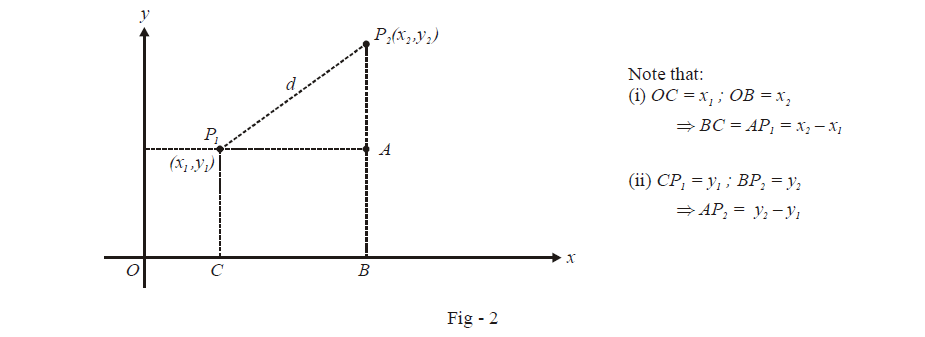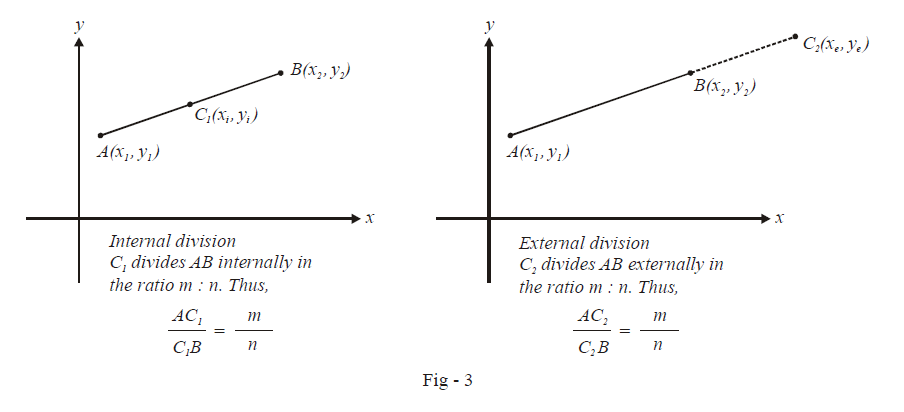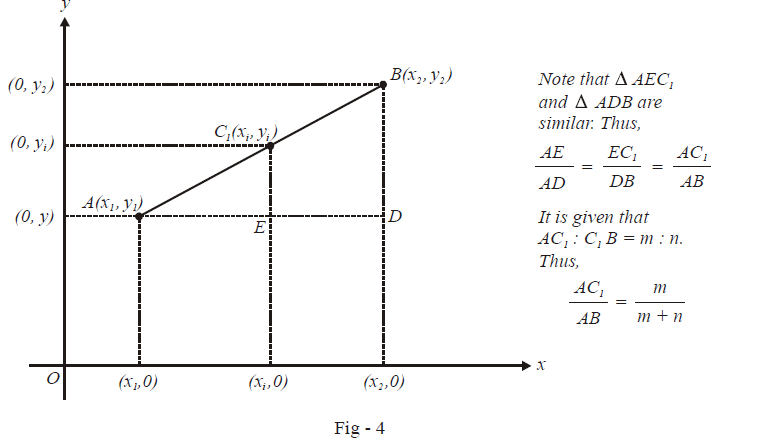# Distance and Section Formulae

Go back to  'Straight Lines'

## Distance and section formula

For a good command over co-ordinate geometry, a lot of results will be required to be memorised since they are encountered so often. For this purpose, each new theorem or result or property that we will encounter in the following pages is discussed in a separate article for ease of reference later.

$$\textbf{Art 1 :} \qquad\boxed{{\text{Distance formula}}}$$

One of the most basic expressions in co-ordinate geometry is that of the distance between two arbitrary points $${P_1}\left( {{x_1},{y_1}} \right)\,\,\,{\text{and}}\,\,{P_2}\left( {{x_2},{y_2}} \right)$$. To obtain the required distance in terms of the co-ordinates of these points, the Pythagoras theorem is employed as described in the figure below:As explained in the figure, the distances AP1 and APhave been obtained. Thus, by the Pythagoras theorem, the distance d is $$\sqrt {AP_1^2 + AP_2^2}$$  or

$\boxed{d = \sqrt {{{\left( {{x_2} - {x_1}} \right)}^2} + {{\left( {{y_2} - {y_1}} \right)}^2}} }$

As a direct consequence of this formula, the distance of an arbitrary point P(x, y) from the origin is $$\sqrt {{x^2} + {y^2}}$$. As an elementary exercise, assume four points anywhere on the co-ordinate plane randomly, and use the distance formula to calculate the distance between each pair of points.

$$\textbf{Art 2 : } \qquad\boxed{{\text{Section formula}}}$$

Suppose that we are given two fixed points in the co-ordinate plane, say $$A\left( {{x_1},{y_1}} \right)\,\,{\text{and}}\,\,B\left( {{x_2},{y_2}} \right)$$. We need to find the co-ordinates of the point C which divides the line segment AB in the ratio m : n. Observe that two such points will exist. Name them Cand C2. One of them will divide the line segment AB internally in the ratio m : n while the other will divide AB in the same ratio externally, as shown in the figure below:Let us find the co-ordinates of C1 using the help of the more detailed figure of internal division below:As described in the figure above,

\begin{align}&\qquad\; \frac{AE}{AD}=\frac{E{{C}_{1}}}{DB}=\frac{m}{m+n} \\\\ & \Rightarrow\quad \frac{{{x}_{i}}-{{x}_{1}}}{{{x}_{2}}-{{x}_{1}}}=\frac{{{y}_{i}}-{{y}_{1}}}{{{y}_{2}}-{{y}_{1}}}=\frac{m}{m+n} \\\\ & \Rightarrow \quad {{x}_{i}}=\frac{m{{x}_{2}}+n{{x}_{1}}}{m+n},{{y}_{i}}=\frac{m{{y}_{2}}+n{{y}_{1}}}{m+n} \end{align}

Thus, the co-ordinates of the point C1 which divides AB internally in the ratio m : n are

$_{Division\,m:n}^{\,\,\,\,\,\,\,Internal}\,\boxed{\frac{{m{x_2} + n{x_1}}}{{m + n}},\frac{{m{y_2} + n{y_1}}}{{m + n}}}$

Using an analogous approach, we can obtain the co-ordinates of the point C2 which divides AB externally in the ratio m : n

$_{Division\,\,m:n}^{\,\,\,\,\,\,\,External}\,\boxed{\frac{{m{x_2} - n{x_1}}}{{m - n}},\frac{{m{y_2} - n{y_1}}}{{m - n}}}$

A particular case of internal division is finding the co-ordinates of the mid-point of AB. Since the mid-point of AB divides the segment AB in the ratio 1 : 1, the co-ordinates of the mid-point will be

$_{\begin{smallmatrix} \, \\ A\equiv \left( {{x}_{1}},{{y}_{1}} \right)\,\,\text{and}\,B\,\equiv \,\,\left( {{x}_{2}},{{y}_{2}} \right) \end{smallmatrix}}^{Mid-point\,of\,AB\,where}\,\boxed{\frac{{{x_1} + {x_2}}}{2},\frac{{{y_1} + {y_2}}}{2}}$

Let us put these rudimentary results to use.

Download SOLVED Practice Questions of Distance and Section Formulae for FREE
Straight Lines
grade 11 | Answers Set 2
Straight Lines
grade 11 | Questions Set 1
Straight Lines
grade 11 | Answers Set 1
Straight Lines
grade 11 | Questions Set 2